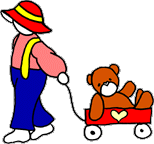Applied Science SECOND GRADE 8 week unit SCIENCE STANDARDS 1. Students should be able to predict dimensions of objects. 2. Students should understand how to verify a prediction. 3. Students should explain the difference between 3 and 2 dimensions. 4. Students should begin distinguishing symmetry. 5. Students should begin distinguishing patterns. 6. Students should begin to understand that geometry helps to describe nature. 7. Students should begin to understand the parameters of physics. 8. Students should be familiar with different forms of energy. 9. Students should recognize that machines make work. 10. Students should identify simple machines. 11. Students should be aware of the different parts of the computer. 12. Students should have learned that different forms of energy are used in our built environment.  |OVERVIEW OF SECOND GRADE SCIENCE AND MATH WEEK 1. PRE: Exploring perception. LAB Experimenting and predicting volume, weight, and length. POST: Estimating and gathering data. WEEK 2. PRE: Comparing and contrasting two and three dimensional objects. LAB: Recognizing and comparing shapes. POST: Exploring unit cells to create patterns. WEEK 3. PRE: Investigating symmetry. LAB: Comparing symmetry in nature. POST: Discovering tessellations. PHYSICS WEEK 4. PRE: Describing the physical world. LAB: Exploring the physics behind toys. POST: Investigating how things work. WEEK 5. PRE: Comparing different forms of energy. LAB: Investigating different forms of energy. POST: Exploring forms of energy. TECHNOLOGY WEEK 6. PRE: Investigating everyday simple machines. LAB: Investigating machines that produce work. POST: Comparing machines that produce energy. WEEK 7. PRE: Exploring technology. LAB: Investigating computer technology. POST: Comparing technologies used in the entertainment industry. BUILT ENVIRONMENT WEEK 8. PRE: Comparing different energy machines. LAB: Investigating how solar energy produces power. POST: Contrasting different forms of energy.

[Back to Applied Science Grid]